﻿ Calculation of Cable Data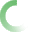The fields with the red border are required.# MEDIUM VOLTAGE SHIELDED CABLE PARAMETER CALCULATOR

The following calculator computes the resistance, inductance, inductive reactance, capacitance, charging current, and surge impedance for medium voltage shielded power cables. This calculator provides a good estimate based on industry standard calculation methods and cable manufacturing data. Typical data, when your specific data is not available, is provided in Table-1 through Table-3.

Calculator-1

Known variables:

Cable Data: Length (feet), Conductor Diameter (inches), Cable Insulation Thickness (inches), Insulation Dielectric Constant, and Operating Frequency (Hertz), and Voltage (kV). When data is not available, use Table-1 through Table-3 for representative values

Cable Installation Detail: Cable Spacing (see figures below) A, B, C, and Correction Factors for Type of Installation (See Table-4)

Conductor Operating Temperature (°C): For cable operation at temperatures other than 20°C, enter expected operating temperature

 Cable Data Approximate Copper Conductor Properties Table-1 Input Cable Operating Voltage (kV): Size Stranding Conductor Diameter (In) Rdc @ 20C    (ohm/1000ft) Rac @ 20 approx. 50/60Hz (ohm/1000ft) Input Cable Length (ft): Input Conductor Diameter (in): Input Cable Insulation thickness (in): 1/0 19 0.373 0.1042 Input Insulation Dielectric Constant: 2/0 19 0.418 0.0827 Input Sheath Thickness (in): 3/0 7 0.464 0.0656 Calculated Cable Radius (in): 4/0 19 0.528 0.0520 Input Cable Operating Frequency (Hz): 250 19 0.574 0.0440 Charging & Capacitance Calculations 350 19 0.679 0.0314 Total Capacitance Calculated: (uF): 500 37 0.814 0.0220 Total Charging Currrent (Amps): 750 61 0.998 0.0147 Charging Vars per Cable (KVAR): 1000 61 1.152 0.0110 Reactance & Inductance Calculatons Cable Spacing Diagram see Figure-1 Cable Insulation Thickness Table-2 Insulation Type Table-3 Cable Spacing A (in): Voltage % Thickness INSULATION TYPE DIELECTRIC CONTSTANT Cable Spacing B (in): XLPE 2.3 Cable Spacing C (in): EPR 3 Correction Factor Installation Type Table-4: 5 100 0.09 PVC 5 Inductance (mH): 5 133 0.09 Reactance (ohms): 8 100 0.115 Correction Factor for Installation Table-4 Resistance Calculation 8 133 0.14 AC Resistance @ 20C: /1000': 15 100 0.175 Air 1 Conductor Operating Temperature (deg. C): 15 133 0.22 Burrial 1 AC Resistance @ Operating Temperature(ohm): 25 100 0.26 Steel Conduit 1.5 Concutor X/R ratio: 25 133 0.32 Al. Conduit 1.2 Surge Impedance Calculation 35 100 0.345 IMT Conduit 1.5 Surge Impedance (ohms): 35 133 0.42 EMT Conduit 1.5 PVC Conduit 1.2 Tray Steel 1.5 Tray Al. 1.2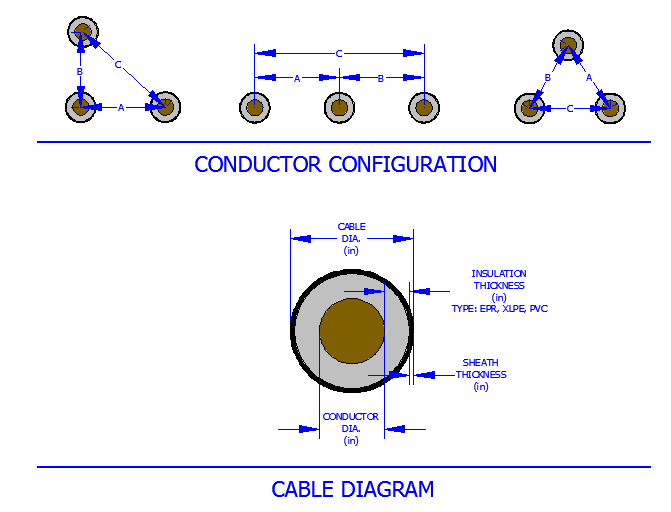Calculated Output: Cable Diameter, Total Capacitance (uF), Total Charging Current (Amps), Charging Vars per phase (kVAR), Charging Reactance (Mohm*1000 ft), Inductance (mH), Reactance (ohms), AC Resistance, X/R ratio, and Surge Impedance (ohms).

Basis of Calculation

Capacitance of Cables, Charging Current, and Charging Reactive Power

The capacitance of a one conductor shielded cable is given by the formula below: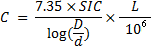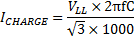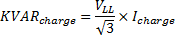Where:
 C = Total capacitance of the cable (microfarads) Icharge = Charging current of the cable SIC = Dielectric constant of the cable insulation (Table-3) D = Diameter over the insulation (in) d = Diameter of the conductor (in) VLL = System operating voltage in (kV) f = System operating frequency (Hz) L = Length of the Cable in Feet Icharge = Charging current (amps) KVARcharge = Single-phase KVAR or charging VARs per cable

Inductance and Reactance of the Cable

The inductance and inductive reactance of three single phase cables is provided by the formulas below. The formulas assume a cable configuration as shown in the figure above. Additionally, since the inductance is dependent upon its material surrounding, use Table-4 to determine an appropriate "K" factor (multiplier) for the inductance.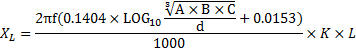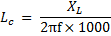Where:
 XL = Conductor inductive reactance (ohms) LC = Cable Inductance (mH) L = Length of the Cable in Feet A, B, C = Spacing per the figure above (in) K = Installation correction factor shown in Table-4 d = Diameter of the conductor (in)

Cable Resistance at Operating Temperature

The resistance of the conductor is provided at 20 deg.C in Table-1. When operating at a different temperature, the resistance varies and is given by the following formula: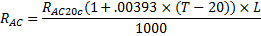Where:
 RAC = AC resistance of the conductor at operating temperature (ohms) RAC20C = AC resistance of the conductor at 20°C (ohm) T = operating temperature of the conductor (°C)

Surge Impedance

The surge impedance of the cable can be calculated with the following formula: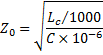Where:
 Zo = Surge Impedance of the Cable (ohm) LC = conductor inductance (mH) C = total capacitance of the cable (microfarads)